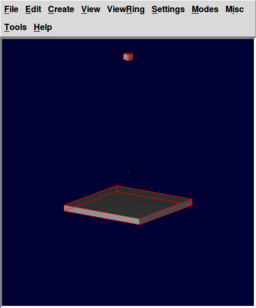# Difference between revisions of "MGED CMD simulate"

BRL-CAD recently integrated a new command into mged, the simulate command, which allows for rigid-body physics simulations. This tutorial demonstrates the usage of the simulate command with a simple scene.Cube falling to the ground plane modeled and rendered in BRL-CAD

First, open the mged interface and create a new database. Next we want to add some geometry to the database. In the command window we will now type the following series of commands:

 ```mged> units m mged> in cube.s rpp -1 1 -1 1 -1 1 mged> r cube.r u cube.s mged> attr set cube.r simulate::type region ```

This will create a cube primitive and a containing region. We will use the region later to apply material appearances. We also set the simulate::type attribute so that the simulate command regards all primitives within this region as one object.

Now we want to add a fixed "ground" object to our simulation. BRL-CAD's simulated gravity acts in the x-y axis, so our ground plane should be parallel to this axis.

 ```mged> in ground.s rpp -15 15 -15 15 -1 1 mged> r ground.r u ground.s mged> attr set ground.r simulate::type region ```

We will also add an attribute specifying that this object's position is fixed:

 ```mged> attr set ground.r simulate::mass 0.0 ```

We can also give the cube an initial angular velocity (linear velocity can be applied in a similar way):

 ```mged> attr set cube.r simulate::angular_velocity <2.0,-1.0,3.0> ```

Now we will add some material appearances to the objects:

 ```mged> mater cube.r "plastic {tr 0.2 re 0.2}" 255 0 0 y mged> mater ground.r "plastic {tr 0.2 re 0.2}" 0 0 255 y ```

To specify our simulated system we will define a combination containing both objects. This combination will be modified in-place during the simulation. We will also change the units of gravity for the simulation to be acceleration in mm/s/s due to the small scale of our model.

 ```mged> comb system.c u cube.r u ground.r mged> attr set system.c simulate::gravity <0,0,-9.80665> ```

We will now select the cube and translate it 50 units "up" along the y-axis.

 ```mged> B system.c mged> oed /system.c/cube.r cube.s mged> tra 0 0 50 mged> accept ```

In order to see what we did so far we should set the view for the geometry we have so far. We will use an "ae 35 15", that means the azimuth angle is at 35 degrees and the elevation at 15 degrees. The angle of elevation of an object as seen by an observer is the angle between the horizontal and the line from the object to the observer's eye (the line of sight).The azimuth of an object is the angular distance along the horizon to the location of the object.We will use "autoview" command to reset the view size and the view center such that all displayed objects are within the view. And then we will do a translation on the Y axis.

 ```mged> ae 35 15 mged> autoview mged> tra 0 10 0 ```

Now we can save this view for later:

 ```mged> saveview ae3515.rt ```

Now we are ready to run the simulate command. To preserve our configuration we will first create a copy of our combination.

 ```mged> cp system.c output.c mged> B output.c mged> simulate output.c 10.0 ```

This will evaluate 10 seconds of simulated physics.

In order to create an animation from your simulation, you will have to run the script below (note that it requires ImageMagick): (in progress)